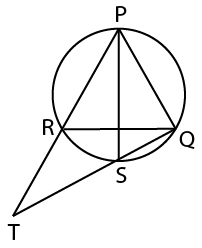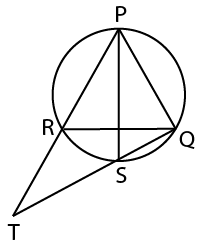Question 91

# In the given figure. PQR is an equilateral triangle and PS is the angle bisector of ∠P. What is the value of RT: RQ?Solution

We haveNow PQR is an equilateral triangle
so Angle PRQ=60
Now therefore angle QRT =180-60=120
Now PS bisects angle RPQ
so angle RPS =30
Now RS is a chord so RPS=RQS =30
So in triangle RTQ
angle RTQ =180-30-120=30
So we get Angle RTQ=RQT
So we can say RT=RQ

• Free SSC Study Material - 18000 Questions
• 230+ SSC previous papers with solutions PDF

##### Jyotimoy Das

3 years, 6 months ago

How?

OR